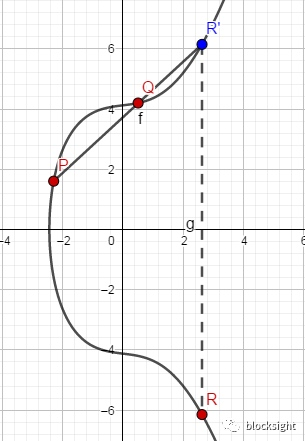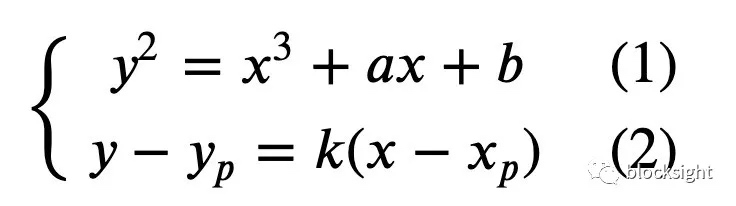## 群与阿贝尔群

1.封闭性：如果 a和b都属于G集合，那么a+b 也属于G;

2.结合律：(a+b)+c=a+(b+c)

3.存在单位元（在二元运算中，单位元指与任意元素运算不改变其值的元素，以实数为例，乘法单位元为1，加法单位元为0）O使得

a+O=O+a=a ;

4.每个元素都存在逆元素，就是说对于任意元素a必存在b使得a+b=O(O是单位元) 。

a+b=b+a

## 椭圆曲线上的群1 𝑂+𝑂=𝑂，对任意的𝑃，有𝑃+𝑂=𝑃；𝑂看做零点

2 𝑃=(𝑥,𝑦) 的负元是关于X中对称的点−𝑃=(𝑥,−𝑦)（而不是关于原点对称），𝑃+(−𝑃)=𝑂，可以看做P与-P连线与椭圆曲线相交于无穷远点

3 计算𝑃点（𝑃≠𝑂）的两倍时，是做该点的切线，再取切线与椭圆曲线交点𝑆关于X轴的对称点−𝑆，也即是2𝑃=𝑃+𝑃=−𝑆，得出2倍值可以递推到若干倍。

## 椭圆曲线群代数运算$x_r=k^2-x_p-x_q$ $y_r=-y_p+k(x_p-x_r)$

$x_r=((3x_p^2+a)/2y_p)^2-2x_p$ $y_r=((3x_p^2+a)/2y_p)(x_p-x_r)-y_p$

• 发表于 2020-04-13 21:28
• 阅读 ( 137 )
• 学分 ( 0 )
• 分类：入门/理论